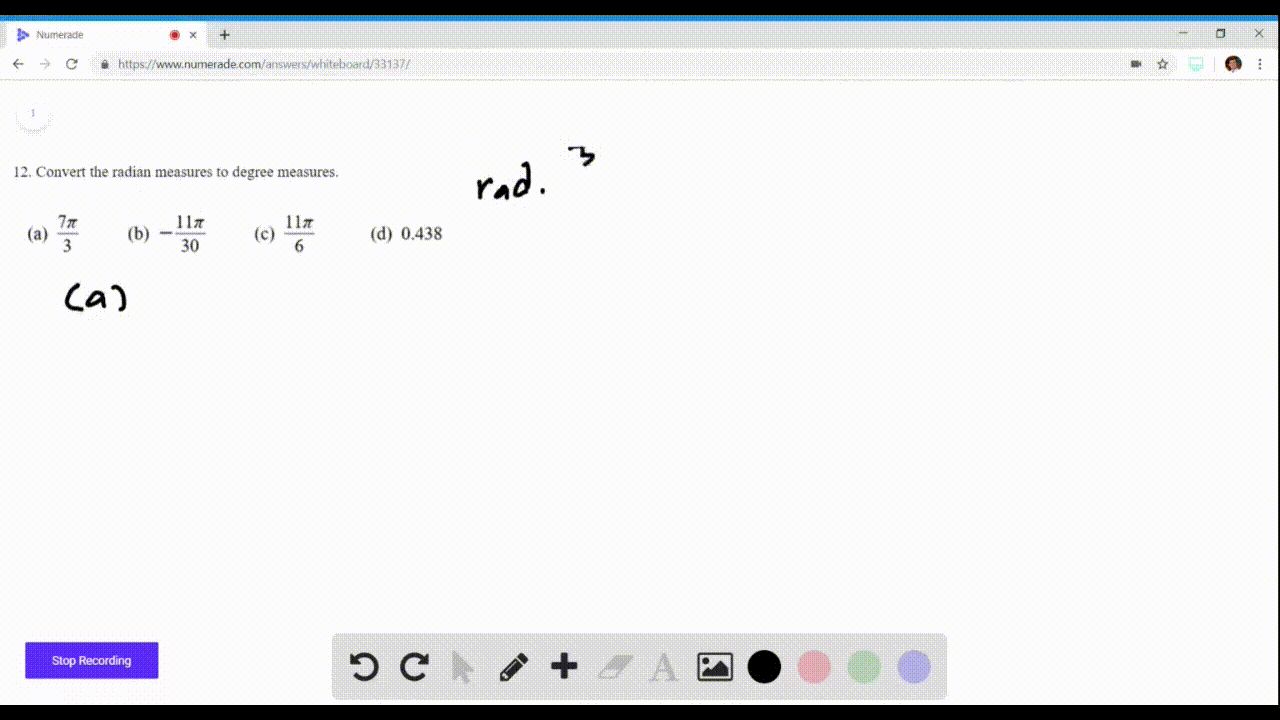🎉 The Study-to-Win Winning Ticket number has been announced! Go to your Tickets dashboard to see if you won! 🎉View Winning Ticket### Radians to Degrees In Exercises 11 and $12$ conve…

ViewUniversity of California, Berkeley
Problem 11

# Radians to Degrees In Exercises 11 and $12,$ convert the radian measure to degree measure.$\begin{array}{lll}{\text { (a) } \frac{3 \pi}{2}} & {\text { (b) } \frac{7 \pi}{6}} & {\text { (c) }-\frac{7 \pi}{12}}\end{array} \quad$ (d) $-2.367$

## Discussion

You must be signed in to discuss.

## Video Transcript

Okay, so we're asked to convert from radiant to the Greek. So what we're gonna do is if we have some radiant degree Alfa, we're gonna multiply it by 1 80 degrees over Pi to get our degrees A We have three pi over too. You multiply that by 1 80 degrees over pipes. Surprise, Cancel. And we're left with 2 72 Report me. We have seven pi over six. Multiply that by 1 80 degrees over pi and we get to 10 degrees. Report. See, we have made of seven ty over 12. Multiply that by 1 80 over pie and we get negative. One of five degrees party. We have negative. 2.367 Multiply that by 1 80 over pie and we get approximately negative Laundry. 5.63 degrees.